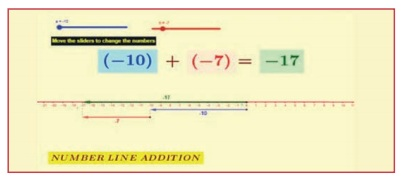Home | | Maths 7th Std | Summary

# Summary

7th Maths : Term 1 Unit 1 : Number System : Summary

Summary

● Integers are a collection of natural numbers, zero and negative numbers.

● The number line gives a visual representation of the set of all integers with positive integers to the right of zero and negative integers to the left of zero.

● The sum of the two positive integers is positive and two negative integers is negative.

● The sum of a positive and a negative integer is the difference of the two numbers in value and has the sign of the greater integer.

● The addition of integers has the closure, commutative and associative properties.

● The product of two positive integers and two negative integers are positive.

● The product of two integers with opposite signs is negative.

● The multiplication of integers has the closure, commutative and associative properties.

● The integer 0 is the additive identity for integers.

● The integer 1 is the multiplicative identity for integers.

ICT Corner

Expected Result is shown in this pictureStep – 1 :

Open the Browser type the URL Link given below (or) Scan the QR Code. GeoGebra work book named “7thstandard” will open. Select Term-1.

Step - 2 :

There are several work sheets for each chapter. Soelect the worksheet “Number System”. In this Number line addition is given. Move the sliders to change the numbers. You can see the answer in green colour arrow.